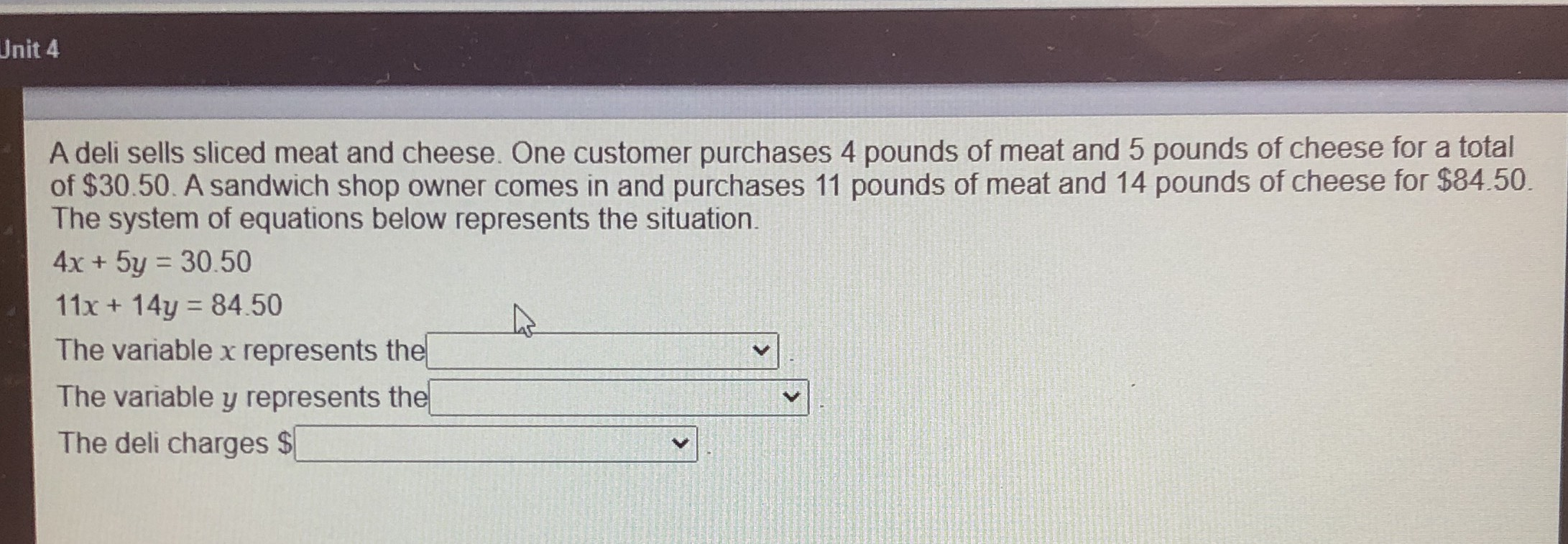### ¿Todavía tienes preguntas de matemáticas?

Pregunte a nuestros tutores expertos
Algebra
PreguntaA deli sells sliced meat and cheese. One customer purchases $$4$$ pounds of meat and $$5$$ pounds of cheese for a total of $$\ 30.50$$ . A sandwich shop owner comes in and purchases $$11$$ pounds of meat and $$14$$ pounds of cheese for $$\ 84.50$$ . The system of equations below represents the situation.

$$4 x + 5 y = 30.50$$

$$11 x + 14 y = 84.50$$

The variable $$x$$ represents the

The variable $$y$$ represents the

The deli charges $$\ \square$$

$$x= 4.5\\ y= 2.5$$